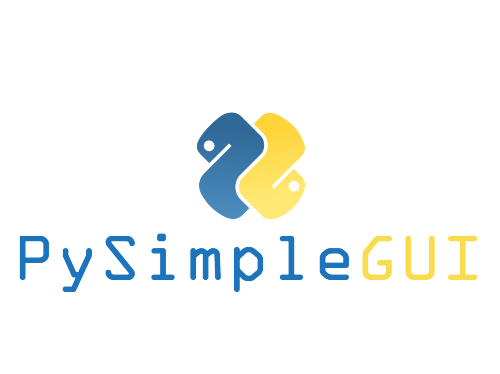# The PySimpleGUI Cookbook

You'll find that starting with a Recipe will give you a big jump-start on creating your custom GUI. Copy and paste one of these Recipes and modify it to match your requirements. Study them to get an idea of what design patterns to follow.

The Recipes in this Cookbook all assume you're running on a Python3 machine. If you are running Python 2.7 then your code will differ by 2 character. Replace the import statement:

``````import PySimpleGUI as sg
``````

with

``````import PySimpleGUI27 as sg
``````

There is a short section in the Readme with instruction on installing PySimpleGUI

If you like this Cookbook, then you'll LOVE the 100+ sample programs that are just like these. You'll find them in the GitHub at http://www.PySimpleGUI.com. These Recipes are simply several of those programs displayed in document format.

# Experimental repl.it Embedded Windows

You'll find a few of these Recipes are running in your browser window using PySimpleGUIWeb. They are included so that you can immediately play around with the SDK before installing one of the PySimpleGUI variants on your computer.

This is a new capability for PySimpleGUI that has only very recently been started. Only a few of the elements are operational using PySimpleGUIWeb. So, be prepared for some bugs. It's got a ways to go, but still seemed valuable to include.

# Copy these design patterns!

All of your PySimpleGUI programs will utilize one of these 2 design patterns depending on the type of window you're implementing. The two types of windows are: 1. One-shot window 2. Persistent window

The one-shot window is one that pops up, collects some data, and then disappears. It is more or less a 'form'.

The "Persistent" window is one that sticks around. With these programs, you loop, reading and processing "events" such as button clicks.

## Pattern 1 - "One-shot Window" - Read int list (The Most Common Pattern)

This will be the most common pattern you'll follow if you are not using an "event loop" (not reading the window multiple times). The window is read and then closes.

Because no "keys" were specified in the window layout, the return values will be a list of values. If a key is present, then the values are a dictionary. See the main readme document or further down in this document for more on these 2 ways of reading window values.

``````import PySimpleGUI as sg

layout = [[sg.Text('My one-shot window.')],
[sg.InputText()],
[sg.Submit(), sg.Cancel()]]

window = sg.Window('Window Title').Layout(layout)

event, values = window.Read()
window.Close()

text_input = values
print(text_input)
``````

## Pattern 2 A - Persistent window (multiple reads using an event loop)

Some of the more advanced programs operate with the window remaining visible on the screen. Input values are collected, but rather than closing the window, it is kept visible acting as a way to both output information to the user and gather input data.

This code will present a window and will print values until the user clicks the exit button or closes window using an X.

Note the `do_not_clear` parameter that is described in the next design pattern.

``````import PySimpleGUI as sg

layout = [[sg.Text('Persistent window')],
[sg.Input(do_not_clear=True)],

window = sg.Window('Window that stays open').Layout(layout)

while True:
event, values = window.Read()
if event is None or event == 'Exit':
break
print(event, values)

window.Close()
``````

## Pattern 2 B - Persistent window (multiple reads using an event loop + updates data in window)

This is a slightly more complex, but maybe more realistic version that reads input from the user and displays that input as text in the window. Your program is likely to be doing both of those activities so this will give you a big jump-start.

Do not worry yet what all of these statements mean. Just copy it so you can begin to play with it, make some changes. Experiment to see how thing work.

A final note... the parameter `do_not_clear` in the input call determines the action of the input field after a button event. If this value is True, the input value remains visible following button clicks. If False, then the input field is CLEARED of whatever was input. If you are building a "Form" type of window with data entry, you likely want False, the default setting (you can remove the parameter completely).

``````import sys
if sys.version_info >= 3:
import PySimpleGUI as sg
else:
import PySimpleGUI27 as sg

layout = [[sg.Text('Your typed chars appear here:'), sg.Text('', key='_OUTPUT_') ],
[sg.Input(do_not_clear=True, key='_IN_')],
[sg.Button('Show'), sg.Button('Exit')]]

window = sg.Window('Window Title').Layout(layout)

while True:                 # Event Loop
event, values = window.Read()
print(event, values)
if event is None or event == 'Exit':
break
if event == 'Show':
# change the "output" element to be the value of "input" element
window.FindElement('_OUTPUT_').Update(values['_IN_'])

window.Close()
``````

# Simple Data Entry - Return Values As List

Same GUI screen except the return values are in a list instead of a dictionary and doesn't have initial values.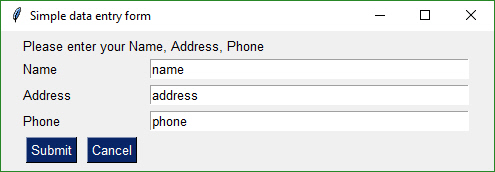``````    import PySimpleGUI as sg

# Very basic window.  Return values as a list

layout = [
[sg.Text('Name', size=(15, 1)), sg.InputText()],
[sg.Text('Address', size=(15, 1)), sg.InputText()],
[sg.Text('Phone', size=(15, 1)), sg.InputText()],
[sg.Submit(), sg.Cancel()]
]

window = sg.Window('Simple data entry window').Layout(layout)
event, values = window.Read()
window.Close()
print(event, values, values, values)
``````

# Simple data entry - Return Values As Dictionary

A simple GUI with default values. Results returned in a dictionary.``````    import PySimpleGUI as sg

# Very basic window.  Return values as a dictionary

layout = [
[sg.Text('Name', size=(15, 1)), sg.InputText('name', key='_NAME_')],
[sg.Text('Phone', size=(15, 1)), sg.InputText('phone', key='_PHONE_')],
[sg.Submit(), sg.Cancel()]
]

window = sg.Window('Simple data entry GUI').Layout(layout)

event, values = window.Read()

window.Close()

print(event, values['_NAME_'], values['_ADDRESS_'], values['_PHONE_'])
``````

## Simple File Browse

Browse for a filename that is populated directly into the user's variable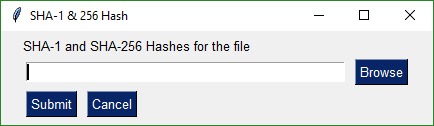``````    import PySimpleGUI as sg

layout = [[sg.Text('SHA-1 and SHA-256 Hashes for the file')],
[sg.InputText(), sg.FileBrowse()],
[sg.Submit(), sg.Cancel()]]

(event, (source_filename,)) = sg.Window('SHA-1 & 256 Hash').Layout(layout ).Read()

print(event, source_filename)
``````

## Add GUI to Front-End of Script

Quickly add a GUI allowing the user to browse for a filename if a filename is not supplied on the command line using this 1-line GUI. It's the best of both worlds.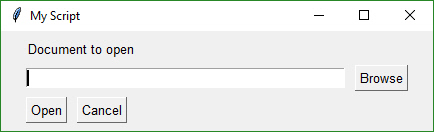``````    import PySimpleGUI as sg
import sys

if len(sys.argv) == 1:
event, (fname,) = sg.Window('My Script').Layout([[sg.Text('Document to open')],
[sg.In(), sg.FileBrowse()],
else:
fname = sys.argv

if not fname:
sg.Popup("Cancel", "No filename supplied")
raise SystemExit("Cancelling: no filename supplied")
print(event, fname)
``````

## Compare 2 Files

Browse to get 2 file names that can be then compared.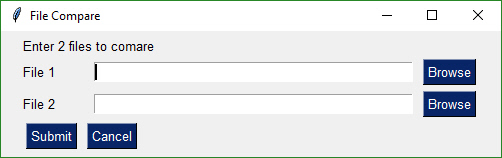``````    import PySimpleGUI as sg

layout = [[sg.Text('Enter 2 files to comare')],
[sg.Text('File 1', size=(8, 1)), sg.InputText(), sg.FileBrowse()],
[sg.Text('File 2', size=(8, 1)), sg.InputText(), sg.FileBrowse()],
[sg.Submit(), sg.Cancel()]]

window = sg.Window('File Compare').Layout(layout)

event, values = window.Read()
window.Close()
print(event, values)
``````

## Nearly All Widgets with Green Color Theme

Example of nearly all of the widgets in a single window. Uses a customized color scheme.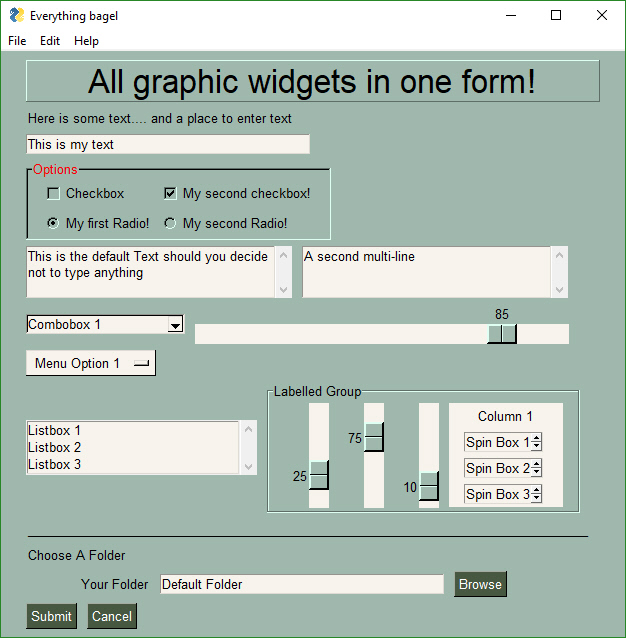``````    #!/usr/bin/env Python3
import PySimpleGUI as sg

sg.ChangeLookAndFeel('GreenTan')

# ------ Menu Definition ------ #
menu_def = [['File', ['Open', 'Save', 'Exit', 'Properties']],
['Edit', ['Paste', ['Special', 'Normal', ], 'Undo'], ],

# ------ Column Definition ------ #
column1 = [[sg.Text('Column 1', background_color='#F7F3EC', justification='center', size=(10, 1))],
[sg.Spin(values=('Spin Box 1', '2', '3'), initial_value='Spin Box 1')],
[sg.Spin(values=('Spin Box 1', '2', '3'), initial_value='Spin Box 2')],
[sg.Spin(values=('Spin Box 1', '2', '3'), initial_value='Spin Box 3')]]

layout = [
[sg.Text('All graphic widgets in one window!', size=(30, 1), justification='center', font=("Helvetica", 25), relief=sg.RELIEF_RIDGE)],
[sg.Text('Here is some text.... and a place to enter text')],
[sg.InputText('This is my text')],
[sg.Frame(layout=[
[sg.Checkbox('Checkbox', size=(10,1)),  sg.Checkbox('My second checkbox!', default=True)],
[sg.Multiline(default_text='This is the default Text should you decide not to type anything', size=(35, 3)),
sg.Multiline(default_text='A second multi-line', size=(35, 3))],
[sg.InputCombo(('Combobox 1', 'Combobox 2'), size=(20, 1)),
sg.Slider(range=(1, 100), orientation='h', size=(34, 20), default_value=85)],
[sg.Listbox(values=('Listbox 1', 'Listbox 2', 'Listbox 3'), size=(30, 3)),
sg.Frame('Labelled Group',[[
sg.Slider(range=(1, 100), orientation='v', size=(5, 20), default_value=25),
sg.Slider(range=(1, 100), orientation='v', size=(5, 20), default_value=75),
sg.Slider(range=(1, 100), orientation='v', size=(5, 20), default_value=10),
sg.Column(column1, background_color='#F7F3EC')]])],
[sg.Text('_'  * 80)],
[sg.Text('Choose A Folder', size=(35, 1))],
[sg.Text('Your Folder', size=(15, 1), auto_size_text=False, justification='right'),
sg.InputText('Default Folder'), sg.FolderBrowse()],
[sg.Submit(tooltip='Click to submit this window'), sg.Cancel()]
]

window = sg.Window('Everything bagel', default_element_size=(40, 1), grab_anywhere=False).Layout(layout)

event, values = window.Read()

sg.Popup('Title',
'The results of the window.',
'The button clicked was "{}"'.format(event),
'The values are', values)

``````

## Non-Blocking Window With Periodic Update

An async Window that has a event read loop. A Text Element is updated periodically with a running timer. Note that `value` is checked for None which indicates the window was closed using X.
Use caution when using windows with a timeout. You should rarely need to use a timeout=0, non-blocking call, so try not to abuse this design pattern.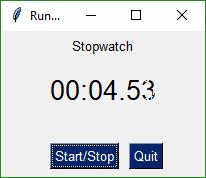``````import PySimpleGUI as sg

layout = [[sg.Text('Stopwatch', size=(20, 2), justification='center')],
[sg.Text('', size=(10, 2), font=('Helvetica', 20), justification='center', key='_OUTPUT_')],
[sg.T(' ' * 5), sg.Button('Start/Stop', focus=True), sg.Quit()]]

window = sg.Window('Running Timer').Layout(layout)

timer_running = True
i = 0
# Event Loop
while True:
i += 1 * (timer_running is True)
event, values = window.Read(timeout=10) # Please try and use a timeout when possible
if event is None or event == 'Quit':  # if user closed the window using X or clicked Quit button
break
elif event == 'Start/Stop':
timer_running = not timer_running
window.FindElement('_OUTPUT_').Update('{:02d}:{:02d}.{:02d}'.format((i // 100) // 60, (i // 100) % 60, i % 100))
``````
``````
``````

## Callback Function Simulation

The architecture of some programs works better with button callbacks instead of handling in-line. While button callbacks are part of the PySimpleGUI implementation, they are not directly exposed to the caller. The way to get the same result as callbacks is to simulate them with a recipe like this one.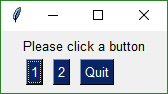``````    import PySimpleGUI as sg

# This design pattern simulates button callbacks
# Note that callbacks are NOT a part of the package's interface to the
# caller intentionally.  The underlying implementation actually does use
# tkinter callbacks.  They are simply hidden from the user.

# The callback functions
def button1():
print('Button 1 callback')

def button2():
print('Button 2 callback')

# Layout the design of the GUI
layout = [[sg.Text('Please click a button', auto_size_text=True)],
[sg.Button('1'), sg.Button('2'), sg.Quit()]]

# Show the Window to the user
window = sg.Window('Button callback example').Layout(layout)

# Event loop. Read buttons, make callbacks
while True:
# Read the Window
event, value = window.Read()
# Take appropriate action based on button
if event == '1':
button1()
elif event == '2':
button2()
elif event =='Quit'  or event is None:
window.Close()
break

# All done!
sg.PopupOK('Done')
``````

## Realtime Buttons (Good For Raspberry Pi)

This recipe implements a remote control interface for a robot. There are 4 directions, forward, reverse, left, right. When a button is clicked, PySimpleGUI immediately returns button events for as long as the buttons is held down. When released, the button events stop. This is an async/non-blocking window.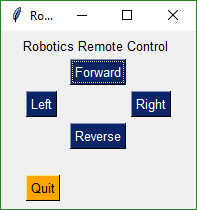``````    import PySimpleGUI as sg

layout = [[sg.Text('Robotics Remote Control')],
[sg.T(' '  * 10), sg.RealtimeButton('Forward')],
[sg.RealtimeButton('Left'), sg.T(' '  * 15), sg.RealtimeButton('Right')],
[sg.T(' '  * 10), sg.RealtimeButton('Reverse')],
[sg.T('')],
[sg.Quit(button_color=('black', 'orange'))]
]

window = sg.Window('Robotics Remote Control', auto_size_text=True).Layout(layout )

#
# Some place later in your code...
# You need to perform a Read or Refresh on your window every now and then or
# else it will appear your program has hung
#
# your program's main loop
while (True):
# This is the code that reads and updates your window
event, values = window.Read(timeout=10)
if event is not None:
print(event)
if event == 'Quit'  or values is None:
break

window.Close()   # Don't forget to close your window!
``````

## OneLineProgressMeter

This recipe shows just how easy it is to add a progress meter to your code.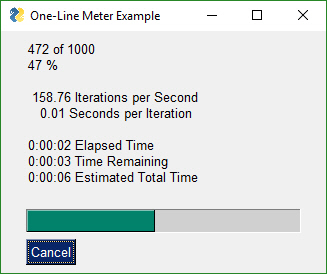``````    import PySimpleGUI as sg

for i in range(1000):
sg.OneLineProgressMeter('One Line Meter Example', i+1, 1000, 'key')
``````

## Button Graphics (Media Player)

Buttons can have PNG of GIF images on them. This Media Player recipe requires 4 images in order to function correctly. The background is set to the same color as the button background so that they blend together.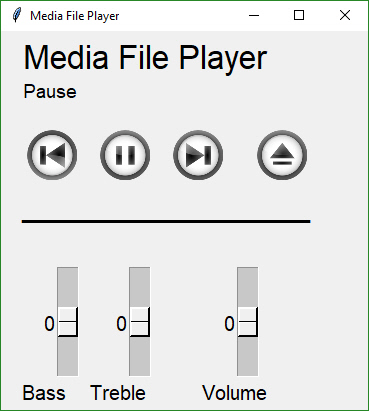``````
#!/usr/bin/env python
import sys
if sys.version_info >= 3:
import PySimpleGUI as sg
else:
import PySimpleGUI27 as sg

#
# An Async Demonstration of a media player
# Uses button images for a super snazzy look
# See how it looks here:
# https://user-images.githubusercontent.com/13696193/43159403-45c9726e-8f50-11e8-9da0-0d272e20c579.jpg
#
def MediaPlayerGUI():
background = '#F0F0F0'
# Set the backgrounds the same as the background on the buttons
sg.SetOptions(background_color=background, element_background_color=background)
# Images are located in a subfolder in the Demo Media Player.py folder
image_pause = './ButtonGraphics/Pause.png'
image_restart = './ButtonGraphics/Restart.png'
image_next = './ButtonGraphics/Next.png'
image_exit = './ButtonGraphics/Exit.png'

# A text element that will be changed to display messages in the GUI

# define layout of the rows
layout= [[sg.Text('Media File Player',size=(17,1), font=("Helvetica", 25))],
[sg.Text('', size=(15, 2), font=("Helvetica", 14), key='output')],
[sg.Button('', button_color=(background,background),
image_filename=image_restart, image_size=(50, 50), image_subsample=2, border_width=0, key='Restart Song'),
sg.Text(' ' * 2),
sg.Button('', button_color=(background,background),
image_filename=image_pause, image_size=(50, 50), image_subsample=2, border_width=0, key='Pause'),
sg.Text(' ' * 2),
sg.Button('', button_color=(background,background), image_filename=image_next, image_size=(50, 50), image_subsample=2, border_width=0, key='Next'),
sg.Text(' ' * 2),
sg.Text(' ' * 2), sg.Button('', button_color=(background,background),
image_filename=image_exit, image_size=(50, 50), image_subsample=2, border_width=0, key='Exit')],
[sg.Text('_'*20)],
[sg.Text(' '*30)],
[
sg.Slider(range=(-10, 10), default_value=0, size=(10, 20), orientation='vertical', font=("Helvetica", 15)),
sg.Text(' ' * 2),
sg.Slider(range=(-10, 10), default_value=0, size=(10, 20), orientation='vertical', font=("Helvetica", 15)),
sg.Text(' ' * 2),
sg.Slider(range=(-10, 10), default_value=0, size=(10, 20), orientation='vertical', font=("Helvetica", 15))],
[sg.Text('   Bass', font=("Helvetica", 15), size=(9, 1)),
sg.Text('Treble', font=("Helvetica", 15), size=(7, 1)),
sg.Text('Volume', font=("Helvetica", 15), size=(7, 1))]
]

# Open a form, note that context manager can't be used generally speaking for async forms
window = sg.Window('Media File Player', auto_size_text=True, default_element_size=(20, 1),
font=("Helvetica", 25)).Layout(layout)
# Our event loop
while(True):
event, values = window.Read(timeout=100)        # Poll every 100 ms
if event == 'Exit' or event is None:
break
# If a button was pressed, display it on the GUI by updating the text element
if event != sg.TIMEOUT_KEY:
window.FindElement('output').Update(event)

MediaPlayerGUI()
``````

## Script Launcher - Persistent Window

This Window doesn't close after button clicks. To achieve this the buttons are specified as `sg.Button` instead of `sg.Button`. The exception to this is the EXIT button. Clicking it will close the window. This program will run commands and display the output in the scrollable window.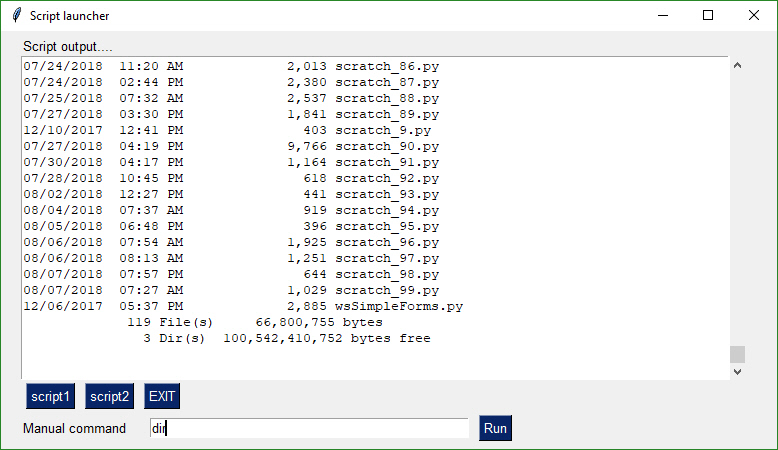``````    import PySimpleGUI as sg
import subprocess

# Please check Demo programs for better examples of launchers
def ExecuteCommandSubprocess(command, *args):
try:
sp = subprocess.Popen([command, *args], shell=True, stdout=subprocess.PIPE, stderr=subprocess.PIPE)
out, err = sp.communicate()
if out:
print(out.decode("utf-8"))
if err:
print(err.decode("utf-8"))
except:
pass

layout = [
[sg.Text('Script output....', size=(40, 1))],
[sg.Output(size=(88, 20))],
[sg.Button('script1'), sg.Button('script2'), sg.Button('EXIT')],
[sg.Text('Manual command', size=(15, 1)), sg.InputText(focus=True), sg.Button('Run', bind_return_key=True)]
]

window = sg.Window('Script launcher').Layout(layout)

# ---===--- Loop taking in user input and using it to call scripts --- #

while True:
(event, value) = window.Read()
if event == 'EXIT'  or event is None:
break # exit button clicked
if event == 'script1':
ExecuteCommandSubprocess('pip', 'list')
elif event == 'script2':
ExecuteCommandSubprocess('python', '--version')
elif event == 'Run':
ExecuteCommandSubprocess(value)
``````

## Launch a Program With a Button

Very simple script that will launch a program as a subprocess. Great for making a desktop launcher toolbar.

``````import subprocess
import PySimpleGUI as sg

CHROME = r"C:\Program Files (x86)\Google\Chrome\Application\chrome.exe"

layout = [  [sg.Text('Text area', key='_TEXT_')],
[sg.Input(do_not_clear=True, key='_URL_')],
[sg.Button('Chrome'), sg.Button('Exit')]]

window = sg.Window('Window Title').Layout(layout)

while True:             # Event Loop
event, values = window.Read()
print(event, values)
if event is None or event == 'Exit':
break
if event == 'Chrome':
sp = subprocess.Popen([CHROME, values['_URL_']], shell=True, stdout=subprocess.PIPE, stderr=subprocess.PIPE)

window.Close()
``````

## Machine Learning GUI

A standard non-blocking GUI with lots of inputs.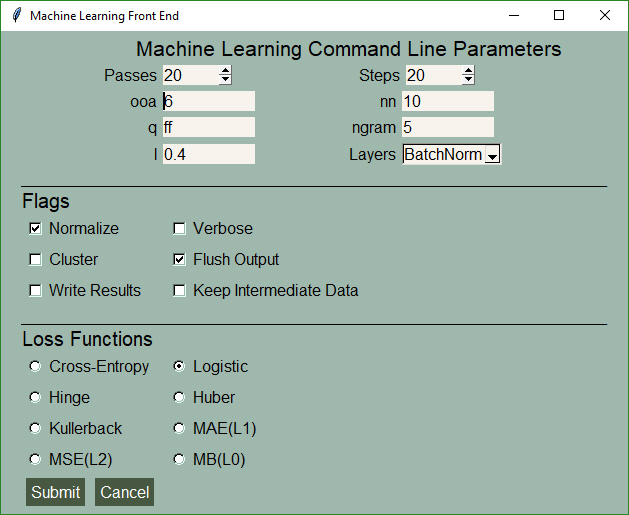``````    import PySimpleGUI as sg

# Green & tan color scheme
sg.ChangeLookAndFeel('GreenTan')

sg.SetOptions(text_justification='right')

layout = [[sg.Text('Machine Learning Command Line Parameters', font=('Helvetica', 16))],
[sg.Text('Passes', size=(15, 1)), sg.Spin(values=[i for i in range(1, 1000)], initial_value=20, size=(6, 1)),
sg.Text('Steps', size=(18, 1)), sg.Spin(values=[i for i in range(1, 1000)], initial_value=20, size=(6, 1))],
[sg.Text('ooa', size=(15, 1)), sg.In(default_text='6', size=(10, 1)), sg.Text('nn', size=(15, 1)),
sg.In(default_text='10', size=(10, 1))],
[sg.Text('q', size=(15, 1)), sg.In(default_text='ff', size=(10, 1)), sg.Text('ngram', size=(15, 1)),
sg.In(default_text='5', size=(10, 1))],
[sg.Text('l', size=(15, 1)), sg.In(default_text='0.4', size=(10, 1)), sg.Text('Layers', size=(15, 1)),
sg.Drop(values=('BatchNorm', 'other'), auto_size_text=True)],
[sg.Text('_'  * 100, size=(65, 1))],
[sg.Text('Flags', font=('Helvetica', 15), justification='left')],
[sg.Checkbox('Normalize', size=(12, 1), default=True), sg.Checkbox('Verbose', size=(20, 1))],
[sg.Checkbox('Cluster', size=(12, 1)), sg.Checkbox('Flush Output', size=(20, 1), default=True)],
[sg.Checkbox('Write Results', size=(12, 1)), sg.Checkbox('Keep Intermediate Data', size=(20, 1))],
[sg.Text('_'  * 100, size=(65, 1))],
[sg.Text('Loss Functions', font=('Helvetica', 15), justification='left')],
[sg.Radio('Cross-Entropy', 'loss', size=(12, 1)), sg.Radio('Logistic', 'loss', default=True, size=(12, 1))],
[sg.Radio('Hinge', 'loss', size=(12, 1)), sg.Radio('Huber', 'loss', size=(12, 1))],
[sg.Radio('Kullerback', 'loss', size=(12, 1)), sg.Radio('MAE(L1)', 'loss', size=(12, 1))],
[sg.Radio('MSE(L2)', 'loss', size=(12, 1)), sg.Radio('MB(L0)', 'loss', size=(12, 1))],
[sg.Submit(), sg.Cancel()]]

window = sg.Window('Machine Learning Front End', font=("Helvetica", 12)).Layout(layout)

event, values = window.Read()
``````

## Custom Progress Meter / Progress Bar

Perhaps you don't want all the statistics that the EasyProgressMeter provides and want to create your own progress bar. Use this recipe to do just that.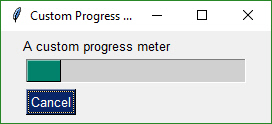``````import PySimpleGUI as sg

# layout the Window
layout = [[sg.Text('A custom progress meter')],
[sg.ProgressBar(1000, orientation='h', size=(20, 20), key='progbar')],
[sg.Cancel()]]

# create the Window
window = sg.Window('Custom Progress Meter').Layout(layout)
# loop that would normally do something useful
for i in range(1000):
# check to see if the cancel button was clicked and exit loop if clicked
event, values = window.Read(timeout=0)
if event == 'Cancel' or event is None:
break
# update bar with loop value +1 so that bar eventually reaches the maximum
window.FindElement('progbar').UpdateBar(i + 1)
# done with loop... need to destroy the window as it's still open
window.Close()
``````

## The One-Line GUI

For those of you into super-compact code, a complete customized GUI can be specified, shown, and received the results using a single line of Python code.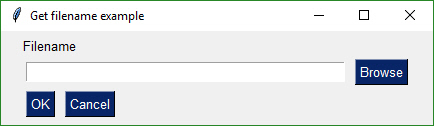``````    import PySimpleGUI as sg

layout = [[sg.Text('Filename')],
[sg.Input(), sg.FileBrowse()],
[sg.OK(), sg.Cancel()]]

event, (number,) = sg.Window('Get filename example').Layout(layout).Read()
``````

you can write this line of code for the exact same result (OK, two lines with the import):

``````    import PySimpleGUI as sg

event, (filename,) = sg.Window('Get filename example').Layout(
[[sg.Text('Filename')], [sg.Input(), sg.FileBrowse()], [sg.OK(), sg.Cancel()]]).Read()
``````

## Multiple Columns

A Column is required when you have a tall element to the left of smaller elements.

In this example, there is a Listbox on the left that is 3 rows high. To the right of it are 3 single rows of text and input. These 3 rows are in a Column Element.

To make it easier to see the Column in the window, the Column background has been shaded blue. The code is wordier than normal due to the blue shading. Each element in the column needs to have the color set to match blue background.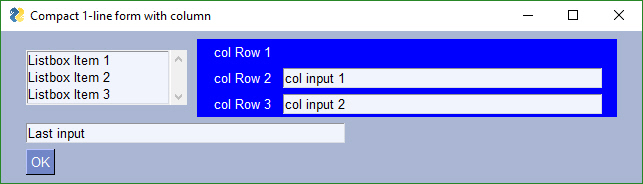``````    import PySimpleGUI as sg

# Demo of how columns work
# GUI has on row 1 a vertical slider followed by a COLUMN with 7 rows
# Prior to the Column element, this layout was not possible
# Columns layouts look identical to GUI layouts, they are a list of lists of elements.

sg.ChangeLookAndFeel('BlueMono')

# Column layout
col = [[sg.Text('col Row 1', text_color='white', background_color='blue')],
[sg.Text('col Row 2', text_color='white', background_color='blue'), sg.Input('col input 1')],
[sg.Text('col Row 3', text_color='white', background_color='blue'), sg.Input('col input 2')]]

layout = [[sg.Listbox(values=('Listbox Item 1', 'Listbox Item 2', 'Listbox Item 3'), select_mode=sg.LISTBOX_SELECT_MODE_MULTIPLE, size=(20,3)), sg.Column(col, background_color='blue')],
[sg.Input('Last input')],
[sg.OK()]]

# Display the Window and get values

event, values = sg.Window('Compact 1-line Window with column').Layout(layout).Read()

sg.Popup(event, values, line_width=200)
``````

## Persistent Window With Text Element Updates

This simple program keep a window open, taking input values until the user terminates the program using the "X" button.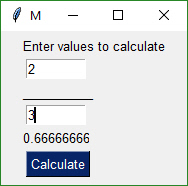```python
import PySimpleGUI as sg

``````layout = [ [sg.Txt('Enter values to calculate')],
[sg.In(size=(8,1), key='numerator')],
[sg.Txt('_'  * 10)],
[sg.In(size=(8,1), key='denominator')],
[sg.Txt('', size=(8,1), key='output')  ],
[sg.Button('Calculate', bind_return_key=True)]]

window = sg.Window('Math').Layout(layout)

while True:
event, values = window.Read()

if event is not None:
try:
numerator = float(values['numerator'])
denominator = float(values['denominator'])
calc = numerator / denominator
except:
calc = 'Invalid'

window.FindElement('output').Update(calc)
else:
break
``````
``````
## One Element Updating  Another - Compound Elements

![image](https://user-images.githubusercontent.com/13696193/49649095-1be40700-f9f6-11e8-981e-f56eb8404ae7.png)

You can easily build "compound elements" in a single like of code.  This recipe shows you how to add a numeric value onto a slider.

```python
import PySimpleGUI as sg

layout = [[sg.Text('Slider Demonstration'), sg.Text('', key='_OUTPUT_')],
[sg.T('0',key='_LEFT_'),
sg.Slider((1,100), key='_SLIDER_', orientation='h', enable_events=True, disable_number_display=True),
sg.T('0', key='_RIGHT_')],
[sg.Button('Show'), sg.Button('Exit')]]

window = sg.Window('Window Title').Layout(layout)

while True:             # Event Loop
event, values = window.Read()
print(event, values)
if event is None or event == 'Exit':
break
window.Element('_LEFT_').Update(values['_SLIDER_'])
window.Element('_RIGHT_').Update(values['_SLIDER_'])

window.Close()
``````

## Multiple Windows

This recipe is a design pattern for multiple windows where the first window is not active while the second window is showing. The first window is hidden to discourage continued interaction.

``````"""
PySimpleGUI The Complete Course Lesson 7 - Multiple Windows"""
import PySimpleGUI as sg

# Design pattern 1 - First window does not remain active

layout = [[ sg.Text('Window 1'),],
[sg.Input(do_not_clear=True)],
[sg.Text('', key='_OUTPUT_')],
[sg.Button('Launch 2')]]

win1 = sg.Window('Window 1').Layout(layout)
win2_active=False
while True:
ev1, vals1 = win1.Read(timeout=100)
if ev1 is None:
break
win1.FindElement('_OUTPUT_').Update(vals1)

if ev1 == 'Launch 2'  and not win2_active:
win2_active = True
win1.Hide()
layout2 = [[sg.Text('Window 2')],       # note must create a layout from scratch every time. No reuse
[sg.Button('Exit')]]

win2 = sg.Window('Window 2').Layout(layout2)
while True:
ev2, vals2 = win2.Read()
if ev2 is None or ev2 == 'Exit':
win2.Close()
win2_active = False
win1.UnHide()
break
``````

## tkinter Canvas Widget

The Canvas Element is one of the few tkinter objects that are directly accessible. The tkinter Canvas widget itself can be retrieved from a Canvas Element like this:

``````    can = sg.Canvas(size=(100,100))
tkcanvas = can.TKCanvas
tkcanvas.create_oval(50, 50, 100, 100)
``````

While it's fun to scribble on a Canvas Widget, try Graph Element makes it a downright pleasant experience. You do not have to worry about the tkinter coordinate system and can instead work in your own coordinate system.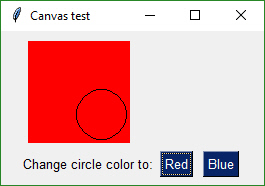```python
import PySimpleGUI as sg

``````layout = [
[sg.Canvas(size=(100, 100), background_color='red', key= 'canvas')],
[sg.T('Change circle color to:'), sg.Button('Red'), sg.Button('Blue')]
]

window = sg.Window('Canvas test')
window.Layout(layout)
window.Finalize()

canvas = window.FindElement('canvas')
cir = canvas.TKCanvas.create_oval(50, 50, 100, 100)

while True:
event, values = window.Read()
if event is None:
break
if event == 'Blue':
canvas.TKCanvas.itemconfig(cir, fill="Blue")
elif event == 'Red':
canvas.TKCanvas.itemconfig(cir, fill="Red")
``````
``````
## Graph Element - drawing circle, rectangle, etc, objects

Just like you can draw on a tkinter widget, you can also draw on a Graph Element.  Graph Elements are easier on the programmer as you get to work in your own coordinate system.

![graph recipe](https://user-images.githubusercontent.com/13696193/45920640-751bb000-be75-11e8-9530-45b71cbae07d.jpg)

```python
import PySimpleGUI as sg

layout = [
[sg.Graph(canvas_size=(400, 400), graph_bottom_left=(0,0), graph_top_right=(400, 400), background_color='red', key='graph')],
[sg.T('Change circle color to:'), sg.Button('Red'), sg.Button('Blue'), sg.Button('Move')]
]

window = sg.Window('Graph test')
window.Layout(layout)
window.Finalize()

graph = window.FindElement('graph')
circle = graph.DrawCircle((75,75), 25, fill_color='black',line_color='white')
point = graph.DrawPoint((75,75), 10, color='green')
oval = graph.DrawOval((25,300), (100,280), fill_color='purple', line_color='purple'  )
rectangle = graph.DrawRectangle((25,300), (100,280), line_color='purple'  )
line = graph.DrawLine((0,0), (100,100))

while True:
event, values = window.Read()
if event is None:
break
if event is 'Blue':
graph.TKCanvas.itemconfig(circle, fill = "Blue")
elif event is 'Red':
graph.TKCanvas.itemconfig(circle, fill = "Red")
elif event is 'Move':
graph.MoveFigure(point, 10,10)
graph.MoveFigure(circle, 10,10)
graph.MoveFigure(oval, 10,10)
graph.MoveFigure(rectangle, 10,10)
``````

## Keypad Touchscreen Entry - Input Element Update

This Recipe implements a Raspberry Pi touchscreen based keypad entry. As the digits are entered using the buttons, the Input Element above it is updated with the input digits.
There are a number of features used in this Recipe including:
Default Element Size
auto_size_buttons
Button
Dictionary Return values
Update of Elements in window (Input, Text)
do_not_clear of Input Elements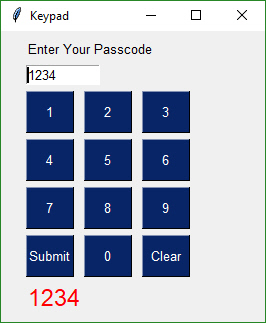```python
import PySimpleGUI as sg

``````# Demonstrates a number of PySimpleGUI features including:
#   Default element size
#   auto_size_buttons
#   Button
#   Dictionary return values
#   Update of elements in window (Text, Input)
#   do_not_clear of Input elements

layout = [[sg.Text('Enter Your Passcode')],
[sg.Input(size=(10, 1), do_not_clear=True, justification='right', key='input')],
[sg.Button('1'), sg.Button('2'), sg.Button('3')],
[sg.Button('4'), sg.Button('5'), sg.Button('6')],
[sg.Button('7'), sg.Button('8'), sg.Button('9')],
[sg.Button('Submit'), sg.Button('0'), sg.Button('Clear')],
[sg.Text('', size=(15, 1), font=('Helvetica', 18), text_color='red', key='out')],
]

window = sg.Window('Keypad', default_button_element_size=(5, 2), auto_size_buttons=False, grab_anywhere=False).Layout(layout)

# Loop forever reading the window's values, updating the Input field
keys_entered = ''
while True:
event, values = window.Read()  # read the window
if event is None:  # if the X button clicked, just exit
break
if event == 'Clear':  # clear keys if clear button
keys_entered = ''
elif event in '1234567890':
keys_entered = values['input']  # get what's been entered so far
keys_entered += event  # add the new digit
elif event == 'Submit':
keys_entered = values['input']
window.FindElement('out').Update(keys_entered)  # output the final string

window.FindElement('input').Update(keys_entered)  # change the window to reflect current key string
``````
``````
<iframe height="800px" width="100%" src="https://repl.it/@PySimpleGUI/Cookbook-Keypad-Touchscreen-Entry?lite=true" scrolling="no" frameborder="no" allowtransparency="true" allowfullscreen="true" sandbox="allow-forms allow-pointer-lock allow-popups allow-same-origin allow-scripts allow-modals"></iframe>

## Animated Matplotlib Graph

Use the Canvas Element to create an animated graph.  The code is a bit tricky to follow, but if you know Matplotlib then this recipe shouldn't be too difficult to copy and modify.

![animated matplotlib](https://user-images.githubusercontent.com/13696193/44640937-91b9ea80-a992-11e8-9c1c-85ae74013679.jpg)

```python
from tkinter import *
from random import randint
import PySimpleGUI as sg
from matplotlib.backends.backend_tkagg import FigureCanvasTkAgg, FigureCanvasAgg
from matplotlib.figure import Figure
import matplotlib.backends.tkagg as tkagg
import tkinter as Tk

fig = Figure()

ax.set_xlabel("X axis")
ax.set_ylabel("Y axis")
ax.grid()

layout = [[sg.Text('Animated Matplotlib', size=(40, 1), justification='center', font='Helvetica 20')],
[sg.Canvas(size=(640, 480), key='canvas')],
[sg.Button('Exit', size=(10, 2), pad=((280, 0), 3), font='Helvetica 14')]]

# create the window and show it without the plot

window = sg.Window('Demo Application - Embedding Matplotlib In PySimpleGUI').Layout(layout)
window.Finalize()  # needed to access the canvas element prior to reading the window

canvas_elem = window.FindElement('canvas')

graph = FigureCanvasTkAgg(fig, master=canvas_elem.TKCanvas)
canvas = canvas_elem.TKCanvas

dpts = [randint(0, 10) for x in range(10000)]
# Our event loop
for i in range(len(dpts)):
event, values = window.Read(timeout=20)
if event == 'Exit' or event is None:
exit(69)

ax.cla()
ax.grid()

ax.plot(range(20), dpts[i:i + 20], color='purple')
graph.draw()
figure_x, figure_y, figure_w, figure_h = fig.bbox.bounds
figure_w, figure_h = int(figure_w), int(figure_h)
photo = Tk.PhotoImage(master=canvas, width=figure_w, height=figure_h)

canvas.create_image(640 / 2, 480 / 2, image=photo)

figure_canvas_agg = FigureCanvasAgg(fig)
figure_canvas_agg.draw()

tkagg.blit(photo, figure_canvas_agg.get_renderer()._renderer, colormode=2)

``````

## Tight Layout with Button States

Saw this example layout written in tkinter and liked it so much I duplicated the interface. It's "tight", clean, and has a nice dark look and feel.

This Recipe also contains code that implements the button interactions so that you'll have a template to build from.

In other GUI frameworks this program would be most likely "event driven" with callback functions being used to communicate button events. The "event loop" would be handled by the GUI engine. If code already existed that used a call-back mechanism, the loop in the example code below could simply call these callback functions directly based on the button text it receives in the window.Read call.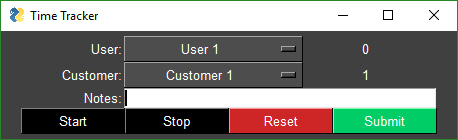``````    import PySimpleGUI as sg
"""
Demonstrates using a "tight" layout with a Dark theme.
Shows how button states can be controlled by a user application.  The program manages the disabled/enabled
states for buttons and changes the text color to show greyed-out (disabled) buttons
"""

sg.ChangeLookAndFeel('Dark')

layout = [[sg.T('User:', pad=((3,0),0)), sg.OptionMenu(values = ('User 1', 'User 2'), size=(20,1)), sg.T('0', size=(8,1))],
[sg.T('Customer:', pad=((3,0),0)), sg.OptionMenu(values=('Customer 1', 'Customer 2'), size=(20,1)), sg.T('1', size=(8,1))],
[sg.T('Notes:', pad=((3,0),0)), sg.In(size=(44,1), background_color='white', text_color='black')],
[sg.Button('Start', button_color=('white', 'black'), key='Start'),
sg.Button('Stop', button_color=('white', 'black'), key='Stop'),
sg.Button('Reset', button_color=('white', 'firebrick3'), key='Reset'),
sg.Button('Submit', button_color=('white', 'springgreen4'), key='Submit')]
]

window = sg.Window("Time Tracker", default_element_size=(12,1), text_justification='r', auto_size_text=False, auto_size_buttons=False,
default_button_element_size=(12,1))
window.Layout(layout)
window.Finalize()
window.FindElement('Stop').Update(disabled=True)
window.FindElement('Reset').Update(disabled=True)
window.FindElement('Submit').Update(disabled=True)
recording = have_data = False
while True:
event, values = window.Read()
print(event)
if event is None:
exit(69)
if event is 'Start':
window.FindElement('Start').Update(disabled=True)
window.FindElement('Stop').Update(disabled=False)
window.FindElement('Reset').Update(disabled=False)
window.FindElement('Submit').Update(disabled=True)
recording = True
elif event is 'Stop'  and recording:
window.FindElement('Stop').Update(disabled=True)
window.FindElement('Start').Update(disabled=False)
window.FindElement('Submit').Update(disabled=False)
recording = False
have_data = True
elif event is 'Reset':
window.FindElement('Stop').Update(disabled=True)
window.FindElement('Start').Update(disabled=False)
window.FindElement('Submit').Update(disabled=True)
window.FindElement('Reset').Update(disabled=False)
recording = False
have_data = False
elif event is 'Submit'  and have_data:
window.FindElement('Stop').Update(disabled=True)
window.FindElement('Start').Update(disabled=False)
window.FindElement('Submit').Update(disabled=True)
window.FindElement('Reset').Update(disabled=False)
recording = False
``````

## Password Protection For Scripts

You get 2 scripts in one.

Use the upper half to generate your hash code. Then paste it into the code in the lower half. Copy and paste lower 1/2 into your code to get password protection for your script without putting the password into your source code.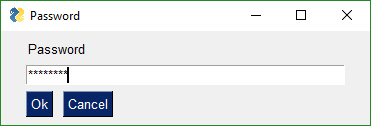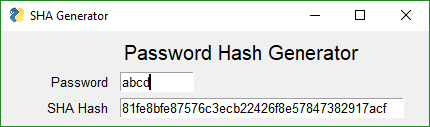``````    import PySimpleGUI as sg
import hashlib

'''
Create a secure login for your scripts without having to include your password    in the program.  Create an SHA1 hash code for your password using the GUI. Paste into variable in final program
1. Choose a password
2. Generate a hash code for your chosen password by running program and entering 'gui' as the password
3. Type password into the GUI
4. Copy and paste hash code Window GUI into variable named login_password_hash
5. Run program again and test your login!
'''

# Use this GUI to get your password's hash code
def HashGeneratorGUI():
layout = [[sg.T('Password Hash Generator', size=(30,1), font='Any 15')],
[sg.T('SHA Hash'), sg.In('', size=(40,1), key='hash')],
]

window = sg.Window('SHA Generator', auto_size_text=False, default_element_size=(10,1),
text_justification='r', return_keyboard_events=True, grab_anywhere=False).Layout(layout)

while True:
event, values = window.Read()
if event is None:
exit(69)

try:
sha1hash = hashlib.sha1()
except:
pass

# ----------------------------- Paste this code into your program / script -----------------------------
# determine if a password matches the secret password by comparing SHA1 hash codes
sha1hash = hashlib.sha1()
if password_hash == hash:
return True
else:
return False

if password == 'gui':                # Remove when pasting into your program
HashGeneratorGUI()               # Remove when pasting into your program
exit(69)                         # Remove when pasting into your program
else:
``````

## Desktop Floating Toolbar

#### Hiding your windows commmand window

For this and the Time & CPU Widgets you may wish to consider using a tool or technique that will hide your Windows Command Prompt window. I recommend the techniques found on this site:

At the moment I'm using the technique that involves wscript and a script named RunNHide.vbs. They are working beautifully. I'm using a hotkey program and launch by using this script with the command "python.exe insert_program_here.py". I guess the next widget should be one that shows all the programs launched this way so you can kill any bad ones. If you don't properly catch the exit button on your window then your while loop is going to keep on working while your window is no longer there so be careful in your code to always have exit explicitly handled.

### Floating toolbar

This is a cool one! (Sorry about the code pastes... I'm working in it)

Impress your friends at what a tool-wizard you are by popping a custom toolbar that you keep in the corner of your screen. It stays on top of all your other windows.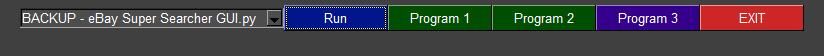You can easily change colors to match your background by changing a couple of parameters in the code.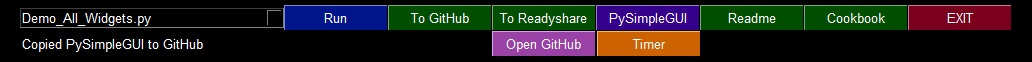``````    import PySimpleGUI as sg
import subprocess
import os
import sys

"""
Demo_Toolbar - A floating toolbar with quick launcher     One cool PySimpleGUI demo. Shows borderless windows, grab_anywhere, tight button layout
You can setup a specific program to launch when a button is clicked, or use the Combobox to select a .py file found in the root folder, and run that file.  """

ROOT_PATH = './'

def Launcher():

def print(line):
window.FindElement('output').Update(line)

sg.ChangeLookAndFeel('Dark')

namesonly = [f for f in os.listdir(ROOT_PATH) if f.endswith('.py') ]

layout =  [[sg.Combo(values=namesonly, size=(35,30), key='demofile'),
sg.Button('Run', button_color=('white', '#00168B')),
sg.Button('Program 1'),
sg.Button('Program 2'),
sg.Button('Program 3', button_color=('white', '#35008B')),
sg.Button('EXIT', button_color=('white','firebrick3'))],
[sg.T('', text_color='white', size=(50,1), key='output')]]

window = sg.Window('Floating Toolbar', no_titlebar=True, keep_on_top=True).Layout(layout)

# ---===--- Loop taking in user input (events) --- #
while True:
(event, value) = window.Read()
if event ==  'EXIT'  or event is None:
break # exit button clicked
if event ==  'Program 1':
print('Run your program 1 here!')
elif event ==  'Program 2':
print('Run your program 2 here!')
elif event ==  'Run':
file = value['demofile']
print('Launching %s'%file)
ExecuteCommandSubprocess('python', os.path.join(ROOT_PATH, file))
else:
print(event)

def ExecuteCommandSubprocess(command, *args, wait=False):
try:
if sys.platwindow == 'linux':
arg_string = ''
for arg in args:
arg_string += ' '  + str(arg)
sp = subprocess.Popen(['python3'  + arg_string, ], shell=True, stdout=subprocess.PIPE, stderr=subprocess.PIPE)
else:
sp = subprocess.Popen([command, list(args)], shell=True, stdout=subprocess.PIPE, stderr=subprocess.PIPE)

if wait:
out, err = sp.communicate()
if out:
print(out.decode("utf-8"))
if err:
print(err.decode("utf-8"))
except: pass

if __name__ == '__main__':
Launcher()
``````

## Desktop Floating Widget - Timer

This is a little widget you can leave running on your desktop. Will hopefully see more of these for things like checking email, checking server pings, displaying system information, dashboards, etc
.
Much of the code is handling the button states in a fancy way. It could be much simpler if you don't change the button text based on state.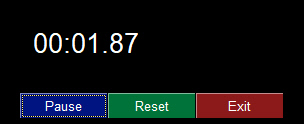``````import sys
if sys.version_info >= 3:
import PySimpleGUI as sg
else:
import PySimpleGUI27 as sg
import time

"""
Timer Desktop Widget Creates a floating timer that is always on top of other windows You move it by grabbing anywhere on the window Good example of how to do a non-blocking, polling program using SimpleGUI Can be used to poll hardware when running on a Pi

While the timer ticks are being generated by PySimpleGUI's "timeout" mechanism, the actual value
of the timer that is displayed comes from the system timer, time.time().  This guarantees an
accurate time value is displayed regardless of the accuracy of the PySimpleGUI timer tick. If
this design were not used, then the time value displayed would slowly drift by the amount of time
it takes to execute the PySimpleGUI read and update calls (not good!)

NOTE - you will get a warning message printed when you exit using exit button.
It will look something like: invalid command name \"1616802625480StopMove\"
"""

# ----------------  Create Form  ----------------
sg.ChangeLookAndFeel('Black')

layout = [[sg.Text('')],
[sg.Text('', size=(8, 2), font=('Helvetica', 20), justification='center', key='text')],
[sg.Button('Pause', key='button', button_color=('white', '#001480')),
sg.Button('Reset', button_color=('white', '#007339'), key='Reset'),
sg.Exit(button_color=('white', 'firebrick4'), key='Exit')]]

window = sg.Window('Running Timer', no_titlebar=True, auto_size_buttons=False, keep_on_top=True, grab_anywhere=True).Layout(layout)

# ----------------  main loop  ----------------
current_time = 0
paused = False
start_time = int(round(time.time() * 100))
while (True):
# --------- Read and update window --------
if not paused:
event, values = window.Read(timeout=10)
current_time = int(round(time.time() * 100)) - start_time
else:
event, values = window.Read()
if event == 'button':
event = window.FindElement(event).GetText()
# --------- Do Button Operations --------
if event is None or event == 'Exit':        # ALWAYS give a way out of program
break
if event is 'Reset':
start_time = int(round(time.time() * 100))
current_time = 0
paused_time = start_time
elif event == 'Pause':
paused = True
paused_time = int(round(time.time() * 100))
element = window.FindElement('button')
element.Update(text='Run')
elif event == 'Run':
paused = False
start_time = start_time + int(round(time.time() * 100)) - paused_time
element = window.FindElement('button')
element.Update(text='Pause')

# --------- Display timer in window --------
window.FindElement('text').Update('{:02d}:{:02d}.{:02d}'.format((current_time // 100) // 60,
(current_time // 100) % 60,
current_time % 100))
``````

## Desktop Floating Widget - CPU Utilization

Like the Timer widget above, this script can be kept running. You will need the package psutil installed in order to run this Recipe.

The spinner changes the number of seconds between reads. Note that you will get an error message printed when exiting because the window does not have have a titlebar. It's a known problem.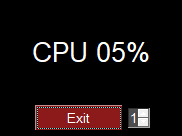``````import PySimpleGUI as sg
import psutil

# ----------------  Create Window  ----------------
sg.ChangeLookAndFeel('Black')
layout = [[sg.Text('')],
[sg.Text('', size=(8, 2), font=('Helvetica', 20), justification='center', key='text')],
[sg.Exit(button_color=('white', 'firebrick4'), pad=((15, 0), 0)),
sg.Spin([x + 1 for x in range(10)], 1, key='spin')]]

window = sg.Window('Running Timer', no_titlebar=True, auto_size_buttons=False, keep_on_top=True,
grab_anywhere=True).Layout(layout)

# ----------------  main loop  ----------------
while (True):
# --------- Read and update window --------
event, values = window.Read(timeout=0)

# --------- Do Button Operations --------
if event is None or event == 'Exit':
break
try:
interval = int(values['spin'])
except:
interval = 1

cpu_percent = psutil.cpu_percent(interval=interval)

# --------- Display timer in window --------

window.FindElement('text').Update(f'CPU {cpu_percent:02.0f}%')

# Broke out of main loop. Close the window.
window.Close()
``````

Menus are nothing more than buttons that live in a menu-bar. When you click on a menu item, you get back a "button" with that menu item's text, just as you would had that text been on a button.

Menu's are defined separately from the GUI window. To add one to your window, simply insert sg.Menu(menu_layout). The menu definition is a list of menu choices and submenus. They are a list of lists. Copy the Recipe and play with it. You'll eventually get when you're looking for.

If you double click the dashed line at the top of the list of choices, that menu will tear off and become a floating toolbar. How cool! To enable this feature, set the parameter `tearoff=True` in your call to `sg.Menu()`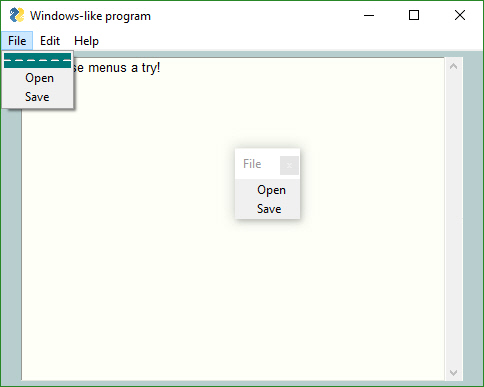``````    import PySimpleGUI as sg

sg.ChangeLookAndFeel('LightGreen')

# ------ Menu Definition ------ #
menu_def = [['File', ['Open', 'Save', 'Exit'  ]],
['Edit', ['Paste', ['Special', 'Normal', ], 'Undo'], ],

# ------ GUI Defintion ------ #
layout = [
[sg.Output(size=(60, 20))]
]

window = sg.Window("Windows-like program", default_element_size=(12, 1), auto_size_text=False, auto_size_buttons=False,
default_button_element_size=(12, 1)).Layout(layout)

# ------ Loop & Process button menu choices ------ #
while True:
event, values = window.Read()
if event == None or event == 'Exit':
break
print('Button = ', event)
# ------ Process menu choices ------ #
if event == 'About...':
elif event == 'Open':
filename = sg.PopupGetFile('file to open', no_window=True)
print(filename)
``````

## Graphing with Graph Element

Use the Graph Element to draw points, lines, circles, rectangles using your coordinate systems rather than the underlying graphics coordinates.

In this example we're defining our graph to be from -100, -100 to +100,+100. That means that zero is in the middle of the drawing. You define this graph description in your call to Graph.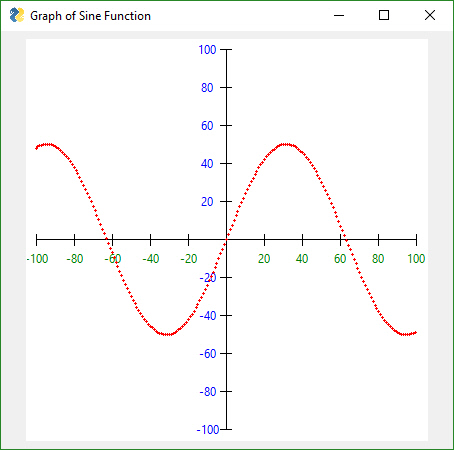``````import math
import PySimpleGUI as sg

layout = [[sg.Graph(canvas_size=(400, 400), graph_bottom_left=(-105,-105), graph_top_right=(105,105), background_color='white', key='graph', tooltip='This is a cool graph!')],]

window = sg.Window('Graph of Sine Function', grab_anywhere=True).Layout(layout).Finalize()
graph = window.FindElement('graph')

# Draw axis
graph.DrawLine((-100,0), (100,0))
graph.DrawLine((0,-100), (0,100))

for x in range(-100, 101, 20):
graph.DrawLine((x,-3), (x,3))
if x != 0:
graph.DrawText( x, (x,-10), color='green')

for y in range(-100, 101, 20):
graph.DrawLine((-3,y), (3,y))
if y != 0:
graph.DrawText( y, (-10,y), color='blue')

# Draw Graph
for x in range(-100,100):
y = math.sin(x/20)*50
graph.DrawCircle((x,y), 1, line_color='red', fill_color='red')

event, values = window.Read()
``````

## Tabs

Tabs bring not only an extra level of sophistication to your window layout, they give you extra room to add more elements. Tabs are one of the 3 container Elements, Elements that hold or contain other Elements. The other two are the Column and Frame Elements.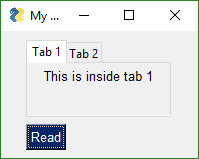``````import PySimpleGUI as sg

tab1_layout =  [[sg.T('This is inside tab 1')]]

tab2_layout = [[sg.T('This is inside tab 2')],
[sg.In(key='in')]]

layout = [[sg.TabGroup([[sg.Tab('Tab 1', tab1_layout, tooltip='tip'), sg.Tab('Tab 2', tab2_layout)]], tooltip='TIP2')],

window = sg.Window('My window with tabs', default_element_size=(12,1)).Layout(layout)

while True:
event, values = window.Read()
print(event,values)
if event is None:           # always,  always give a way out!
break
``````

## Creating a Windows .EXE File

It's possible to create a single .EXE file that can be distributed to Windows users. There is no requirement to install the Python interpreter on the PC you wish to run it on. Everything it needs is in the one EXE file, assuming you're running a somewhat up to date version of Windows.

Installation of the packages, you'll need to install PySimpleGUI and PyInstaller (you need to install only once)

``````pip install PySimpleGUI
pip install PyInstaller
``````

To create your EXE file from your program that uses PySimpleGUI, `my_program.py`, enter this command in your Windows command prompt:

``````pyinstaller -wF my_program.py
``````

You will be left with a single file, `my_program.exe`, located in a folder named `dist` under the folder where you executed the `pyinstaller` command.

That's all... Run your `my_program.exe` file on the Windows machine of your choosing.

"It's just that easy."

(famous last words that screw up just about anything being referenced)

Your EXE file should run without creating a "shell window". Only the GUI window should show up on your taskbar.